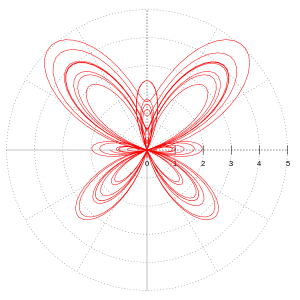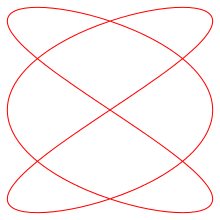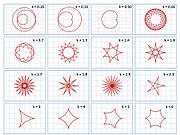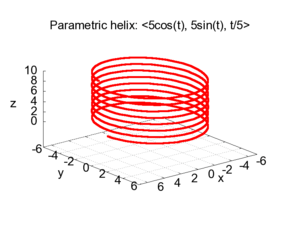# Parametric equationThe butterfly curve can be defined by parametric equations of x and y.

In mathematics, parametric equations define a group of quantities as functions of one or more independent variables called parameters. Parametric equations are commonly used to express the coordinates of the points that make up a geometric object such as a curve or surface, in which case the equations are collectively called a parametric representation or parameterization of the object. For example, the equationsform a parametric representation of the unit circle, where t is the parameter.

In addition to curves and surfaces, parametric equations can describe manifolds and algebraic varieties of higher dimension, with the number of parameters being equal to the dimension of the manifold or variety, and the number of equations being equal to the dimension of the space in which the manifold or variety is considered (for curves the dimension is one and one parameter is used, for surfaces dimension two and two parameters, etc.).

Parametric equations are commonly used in kinematics, where the trajectory of an object is represented by equations depending on time as the parameter. Because of this application, a single parameter is often labeled t; however, parameters can represent other physical quantities (such as geometric variables) or can be selected arbitrarily for convenience. Parameterizations are non-unique; more than one set of parametric equations can specify the same curve.

## Applications

### Kinematics

In kinematics, objects' paths through space are commonly described as parametric curves, with each spatial coordinate depending explicitly on an independent parameter (usually time). Used in this way, the set of parametric equations for the object's coordinates collectively constitute a vector-valued function for position. Such parametric curves can then be integrated and differentiated termwise. Thus, if a particle's position is described parametrically asthen its velocity can be found asand its acceleration as.

### Computer-aided design

Another important use of parametric equations is in the field of computer-aided design (CAD). For example, consider the following three representations, all of which are commonly used to describe planar curves.

Type Form Example Description
1. ExplicitLine
2. ImplicitCircle
3. Parametric;Line

Circle

The first two types are known as analytic, or non-parametric, representations of curves; when compared to parametric representations for use in CAD applications, non-parametric representations have shortcomings. In particular, the non-parametric representation depends on the choice of the coordinate system and does not lend itself well to geometric transformations, such as rotations, translations, and scaling; non-parametric representations therefore make it more difficult to generate points on a curve. These problems can be addressed by rewriting the non-parametric equations in parametric form.

### Integer geometry

Numerous problems in integer geometry can be solved using parametric equations. A classical such solution is Euclid's parametrization of right triangles such that the lengths of their sides a, b and their hypotenuse c are coprime integers. As a and b are not both even (otherwise a, b and c would not be coprime), one may exchange them for having a even, and the parameterization is thenwhere m and n are positive coprime integers that are not both odd.

By multiplying a, b and c by an arbitrary positive integer, one gets a parametrization of all right triangles whose three sides have integer lengths.

## Implicitization

Converting a set of parametric equations to a single equation involves eliminating the variablefrom the simultaneous equationsThis process is called implicitization. If one of these equations can be solved for t, the expression obtained can be substituted into the other equation to obtain an equation involving x and y only.

If the parametrization is given by rational functionswhere p, q, r are set-wise coprime polynomials, a resultant computation allows to implicitize. More precisely, the implicit equation is the resultant with respect to t of xr(t) – p(t) and yr(t) – q(t)

In higher dimension (either more than two coordinates of more than one parameter), the implicitization of rational parametric equations may by done with Gröbner basis computation; see Gröbner basis § Implicitization in higher dimension.

In some cases there is no single equation in closed form that is equivalent to the parametric equations.

To take the example of the circle of radius a above, the parametric equationscan be implicitized in terms of x and y by way of the Pythagorean trigonometric identity:

Asandwe getand thuswhich is the standard equation of a circle centered at the origin.

## Examples in two dimensions

### Parabola

The simplest equation for a parabola,can be (trivially) parameterized by using a free parameter t, and setting### Explicit equations

More generally, any curve given by an explicit equationcan be (trivially) parameterized by using a free parameter t, and setting### Circle

A more sophisticated example is the following. Consider the unit circle which is described by the ordinary (Cartesian) equationThis equation can be parameterized as follows:With the Cartesian equation it is easier to check whether a point lies on the circle or not. With the parametric version it is easier to obtain points on a plot.

In some contexts, parametric equations involving only rational functions (that is fractions of two polynomials) are preferred, if they exist. In the case of the circle, such a rational parameterization isWith this parametric equation, the point (-1, 0) is not represented by a real value of t, but by the limit of x and y when t tends to infinity.

### Ellipse

An ellipse in canonical position (center at origin, major axis along the X-axis) with semi-axes a and b can be represented parametrically asAn ellipse in general position can be expressed asas the parameter t varies from 0 to 2π. Hereis the center of the ellipse, andis the angle between the-axis and the major axis of the ellipse.

Both parametrizations may be made rational by using tangent half-angle formula and setting### Lissajous CurveA Lissajous curve whereand.

A Lissajous curve is similar to an ellipse, but the x and y sinusoids are not in phase. In canonical position, a Lissajous curve is given bywhereandare constants describing the number of lobes of the figure.

### Hyperbola

An east-west opening hyperbola can be represented parametrically byor, rationallyA north-south opening hyperbola can be represented parametrically asIn all formulae (h,k) are the center coordinates of the hyperbola, a is the length of the semi-major axis, and b is the length of the semi-minor axis.

### Hypotrochoid

A hypotrochoid is a curve traced by a point attached to a circle of radius r rolling around the inside of a fixed circle of radius R, where the point is at a distance d from the center of the interior circle.

The parametric equations for the hypotrochoids are:### Some sophisticated functions

Other examples are shown:Several graphs by variation of k## Examples in three dimensions

### HelixParametric helix

Parametric equations are convenient for describing curves in higher-dimensional spaces. For example:describes a three-dimensional curve, the helix, with a radius of a and rising by 2πb units per turn. Note that the equations are identical in the plane to those for a circle. Such expressions as the one above are commonly written aswhere r is a three-dimensional vector.

### Parametric surfaces

Main article: Parametric surface

A torus with major radius R and minor radius r may be defined parametrically aswhere the two parameters t and u both vary between 0 and 2π.

As u varies from 0 to 2π the point on the surface moves about a short circle passing through the hole in the torus. As t varies from 0 to 2π the point on the surface moves about a long circle around the hole in the torus.

## Notes

1. Weisstein, Eric W. "Parametric Equations". MathWorld.
2. Thomas, George B.; Finney, Ross L. (1979). Calculus and Analytic Geometry (fifth ed.). Addison-Wesley. p. 91.
3. Weisstein, Eric W. "Parameterization". MathWorld.
4. Spitzbart, Abraham (1975). Calculus with Analytic Geometry. Gleview, IL: Scott, Foresman and Company. ISBN 0-673-07907-4. Retrieved August 30, 2015.
5. Stewart, James (2003). Calculus (5th ed.). Belmont, CA: Thomson Learning, Inc. pp. 687–689. ISBN 0-534-39339-X.
6. Shah, Jami J.; Martti Mantyla (1995). Parametric and feature-based CAD/CAM: concepts, techniques, and applications. New York, NY: John Wiley & Sons, Inc. pp. 29–31. ISBN 0-471-00214-3.
7. See "Equation form and Parametric form conversion" for more information on converting from a series of parametric equations to single function.